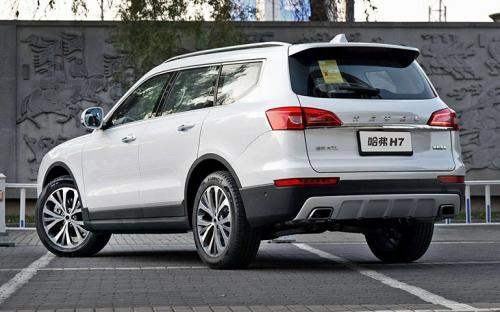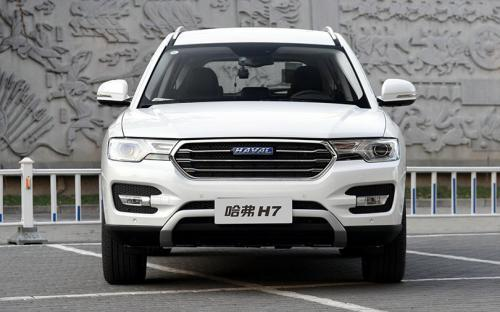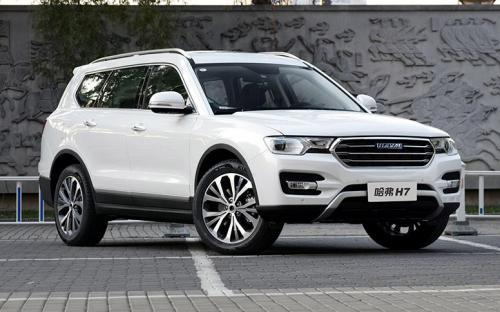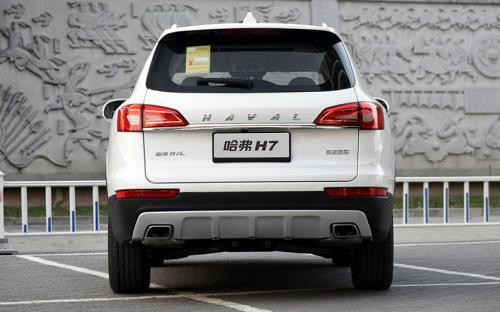### 哈弗 哈弗H72017款最低售价：16.28 万元起

4900(mm)1925(mm)1785(mm)##### 配置亮点：
• 胎压监测装置

• ISOFIX儿童座椅接口

• 车身稳定控制(ESC/ESP/DSC等)

• 电动天窗

• 定速巡航

• 后倒车雷达

• 真皮座椅

• GPS导航系统

• 氙气大灯

• 后视镜加热

• 提交
2017款 红标H7 2.0T 自动精英型 (137张)
• 2017款 红标H7 2.0T 自动精英型 (137张)
• 2017款 红标H7 2.0T 自动尊贵型 (148张)
• 哈弗 哈弗H7 绕车实拍• 哈弗 哈弗H7 在售车型

排量 车型 厂商指导价 本地最低报价 购车工具
L
17.18万
T
16.28万
2.0T
16.28万

### 哈弗 哈弗H7 动力加速

哈弗H7 0-100公里加速时间分布在 0.0--秒 属于 超跑级

动力级别 加速时间 车型

哈弗 哈弗H7 视频

哈弗 哈弗H7 新闻资讯

# 哈弗H7告诉你 这才是哈弗家族最好的车

汽车导购 超过12223次关注

低调是一种人生大智慧，而哈弗H7为我们真正诠释了这一人生哲理。它是哈弗家族最好的车，科技领先、颜值气质出众、动力惊人，但哈弗H7保持低调不张扬。

# 2018款哈弗H7：世界地球日 论一辆SUV的环保修养

国产新车 超过13488次关注

“世界地球日”便是人们专为世界环境保护而设立的节日，倡导人们节能环保，绿色生活。发展到如今，世界地球日的影响已发展至全球192个国家，每年有超过10亿人参与其...

# 哈弗H7L蓝标/H7红标上市 售价16.28-19.38万

国产新车 超过8375次关注

2016年11月11日，哈弗正式公布了旗下H7L蓝标版和H7红标版两个系列车型的售价，其中哈弗H7L蓝标版将提供精英型、豪华型和尊贵型三款配置，H7红标版共提供精英型、豪...

# 哈弗H7L蓝标版/H7红标版配置信息曝光

国产新车 超过8930次关注

日前，我们获得了一组哈弗H7L蓝标版和H7红标版的配置信息，两款车的动力系统将延续现款H7蓝标版的2.0T+6速双离合动力组合。根据之前报道，新车有望在11月11日上市。

# 售18.38-23.18万元 新款哈弗H8正式上市

上市新车 超过7834次关注

新车将比现款车型增加了电子档把等配置，同时动力上提供2.0T汽油和2.0T柴油两款发动机，传动方面升级为来自采埃孚的8速自动变速箱。

# 2016北京车展 哈弗蓝标H7售14.98万起

上市新车 超过8197次关注

在本届北京车展上，哈弗为我们公布了蓝标H7的售价，H7搭载2.0T发动机，新车共推出3款车型，售价为14.98-16.98万元。红标H7将随后上市，蓝标H7L也将在年底上市。

# 哈弗H7/全新概念车等 哈弗车展阵容发布

国产新车 超过8260次关注

哈弗汽车正式公布了参加2016北京车展的展车阵容，其中，全新中型SUV哈弗H7将正式公布官方指导售价，同时，定位为紧凑型SUV和中型SUV的两款全新概念车也将正式亮相。

# 哈弗H7将于1月底上市 长城未来规划曝光

国产新车 超过8342次关注

据官方获悉，长城未来汽车的规划曝光，哈弗H7和H7L将1月底上市，到2016年底之前还会陆续上市10余款新车，之后在2017年推出哈弗品牌的插电式混合动力及纯电动SUV车型...

猜你喜欢

﻿
• 快速找车
• 选择品牌
• 选择品牌
• A  奥迪
• A  阿斯顿·马丁
• A  阿尔法·罗密欧
• B  宝沃
• B  布加迪
• B  巴博斯
• B  保时捷
• B  宾利
• B  奔驰
• B  宝马
• B  本田
• B  别克
• B  标致
• B  比亚迪
• B  宝骏
• B  北汽制造
• B  北汽新能源
• B  北汽幻速
• B  北汽威旺
• B  北京汽车
• B  奔腾
• B  北汽绅宝
• C  长安
• C  长安商用
• C  长城
• C  昌河
• D  大众
• D  道奇
• D  DS
• D  东南
• D  东风风神
• D  东风风行
• D  东风小康
• D  东风风度
• D  东风
• F  福特
• F  丰田
• F  菲亚特
• F  法拉利
• F  福田
• F  福迪
• F  福汽启腾
• G  观致
• G  广汽传祺
• G  广汽吉奥
• G  GMC
• H  红旗
• H  汉腾汽车
• H  哈弗
• H  哈飞
• H  海格
• H  海马
• H  华颂
• H  黄海
• H  华泰
• H  恒天
• J  吉利汽车
• J  捷豹
• J  Jeep
• J  江淮
• J  江铃
• J  金杯
• J  九龙
• J  金旅
• K  凯翼
• K  凯迪拉克
• K  克莱斯勒
• K  科尼塞克
• K  卡威
• K  开瑞
• L  路虎
• L  林肯
• L  劳斯莱斯
• L  兰博基尼
• L  雷克萨斯
• L  铃木
• L  雷诺
• L  理念
• L  力帆
• L  莲花汽车
• L  猎豹
• L  路特斯
• L  陆风
• M  马自达
• M  MG
• M  MINI
• M  玛莎拉蒂
• M  摩根
• M  迈凯轮
• N  纳智捷
• O  欧宝
• O  讴歌
• O  欧朗
• Q  奇瑞
• Q  起亚
• Q  启辰
• R  日产
• R  荣威
• R  瑞麒
• S  三菱
• S  斯威汽车
• S  萨博
• S  smart
• S  斯柯达
• S  斯巴鲁
• S  思铭
• S  双龙
• S  上汽大通
• S  双环
• T  特斯拉
• T  腾势
• W  沃尔沃
• W  五菱汽车
• W  五十铃
• W  威兹曼
• W  威麟
• X  现代
• X  雪佛兰
• X  雪铁龙
• X  西雅特
• Y  一汽
• Y  英菲尼迪
• Y  英致
• Y  依维柯
• Y  野马汽车
• Y  永源
• Z  众泰
• Z  中华
• Z  中兴
• Z  知豆
• 选择车系
• 选择车系
• 车型对比
• 选择品牌
• 选择品牌
• A  奥迪
• A  阿斯顿·马丁
• A  阿尔法·罗密欧
• B  宝沃
• B  布加迪
• B  巴博斯
• B  保时捷
• B  宾利
• B  奔驰
• B  宝马
• B  本田
• B  别克
• B  标致
• B  比亚迪
• B  宝骏
• B  北汽制造
• B  北汽新能源
• B  北汽幻速
• B  北汽威旺
• B  北京汽车
• B  奔腾
• B  北汽绅宝
• C  长安
• C  长安商用
• C  长城
• C  昌河
• D  大众
• D  道奇
• D  DS
• D  东南
• D  东风风神
• D  东风风行
• D  东风小康
• D  东风风度
• D  东风
• F  福特
• F  丰田
• F  菲亚特
• F  法拉利
• F  福田
• F  福迪
• F  福汽启腾
• G  观致
• G  广汽传祺
• G  广汽吉奥
• G  GMC
• H  红旗
• H  汉腾汽车
• H  哈弗
• H  哈飞
• H  海格
• H  海马
• H  华颂
• H  黄海
• H  华泰
• H  恒天
• J  吉利汽车
• J  捷豹
• J  Jeep
• J  江淮
• J  江铃
• J  金杯
• J  九龙
• J  金旅
• K  凯翼
• K  凯迪拉克
• K  克莱斯勒
• K  科尼塞克
• K  卡威
• K  开瑞
• L  路虎
• L  林肯
• L  劳斯莱斯
• L  兰博基尼
• L  雷克萨斯
• L  铃木
• L  雷诺
• L  理念
• L  力帆
• L  莲花汽车
• L  猎豹
• L  路特斯
• L  陆风
• M  马自达
• M  MG
• M  MINI
• M  玛莎拉蒂
• M  摩根
• M  迈凯轮
• N  纳智捷
• O  欧宝
• O  讴歌
• O  欧朗
• Q  奇瑞
• Q  起亚
• Q  启辰
• R  日产
• R  荣威
• R  瑞麒
• S  三菱
• S  斯威汽车
• S  萨博
• S  smart
• S  斯柯达
• S  斯巴鲁
• S  思铭
• S  双龙
• S  上汽大通
• S  双环
• T  特斯拉
• T  腾势
• W  沃尔沃
• W  五菱汽车
• W  五十铃
• W  威兹曼
• W  威麟
• X  现代
• X  雪佛兰
• X  雪铁龙
• X  西雅特
• Y  一汽
• Y  英菲尼迪
• Y  英致
• Y  依维柯
• Y  野马汽车
• Y  永源
• Z  众泰
• Z  中华
• Z  中兴
• Z  知豆
• 选择车系
• 选择车系
• 选择车型
• 选择车型
• 意见反馈